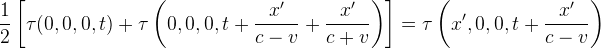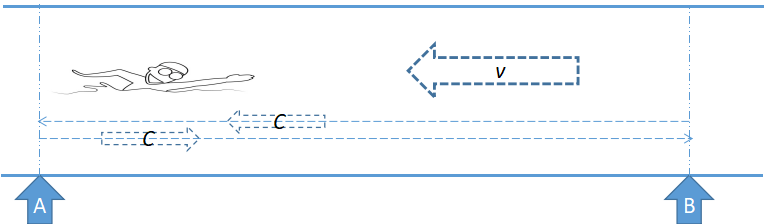## What is Special Relativity (5)

If we followed the same path, then why is the solution of Special Relativity unique, while we have several solutions?

We know that Speed=Distance/Time.
To get a bigger speed, we can make the distance bigger, or make the time smaller.
Mathematically, we have one equation with two variables, so it will have numerous solutions. To get a unique solution, one other restriction is needed.

For each of our three solutions, we added one of these restrictions respectively: keep the distance unchanged, keep the time unchanged, or change both by the same extent.

The other restriction used by Special Relativity is the clock synchronization mechanism proposed by Einstein.

* The meaning of the starting equation of Special Relativity:
half of the round-trip time = upstream time
For a perfect match, the water flowing direction in our graph has to be reversed.